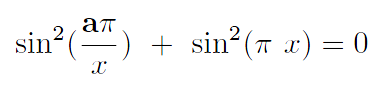Sinpixa
documented 20130707www.heteroclinic.net

Zhikai's Composite Number Equation
In November 2010, Zhikai worked out an equation he hoped may be used to factor composite numbers. It didn't turn out some easy and immediate results. Now, Zhikai is not longer in the area of mathematics, neither pure math nor computational. But Zhikai would like to practice Latex and compare it to similar products. At the same time, Zhikai is still an active mathematical thinker in computer science methodologies.Now we have a smooth function. It grants us the possibility to apply various numerical techniques to solve the equation. If the Theorem \ref{equ1} can provide any result meaningful, we will gladly see that number theory and analysis wed again through the beautiful, curvatual $\pi$

Project Sinpixa 1-1
Sinpixa is the project code name for Equation where a is positive integer greater than 1. Euq.1.1 expresses itself very difficult to be solved. Here, rather than seeking a solution, we would like to start an investigation upon the graphical presentation of this equation. For 1-manifold a+ and a- are the homotopies of Function1.2, so do a + 1 and a - 1. Will the solutions of a - 1 or a + 1 give a kind of bound of the solutions of Euq.1.1, or mutually, or not at all?
Project Sinpixa 1-2
The Beginning
First, we compute and visualize function
We computed two hundred and one points equally paced along x-axis. These points form the following diagram Fig(1). We can use qt-plaut to read the at- tached data file s.sinpixa6'. The following are the qt-plaut instructions.
add a solution' open s.sinpixa6'.
click Scene tools', in drop box Uploaded solutions' choose 2d' at Plotting di- mensions'
click Construct a manifold'.
Scroll down, in Manifold settings' choose hide'.
Scroll down, in Show orbits' choose all'.
In Put marks' click Read', locate and select file
`sinpixa6.lbl'. The diagram meets our expectation in general.We can also observe a "symetric"- like behavior about x equal to square root of 6.
Project Sinpixa 1-3
More Diagrams
We compute and visualize more diagrams, e.g., a = 7, a = 8, a = 9, and a = 143. 143 is the product of 11 and 13. Comparing Fig (5) and Fig (6), Fig (5) demonstrates an interesting behavior we can not explain. Or it is just a coincidence.
In Fig (7), we adjust the function as, it shows better how wave sin^2 \pi x and sin^2 \pi a/x interacts. One tries to touch down the x-axis, the other just prevent it from happening unless an exact coupling.